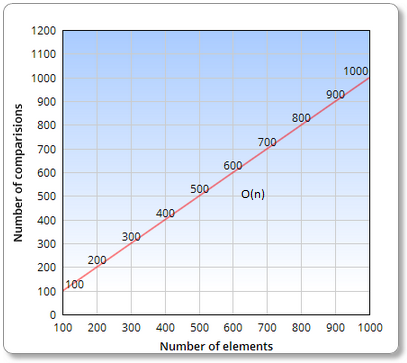### Linear Search in java

Linear Search is most simplest of the way to searching element in array but consumes so much time. As an alternative we can use Binary Search which is much faster as compared to Linear Search.

We’ll be using the LinearSearchArray class to encapsulate the array and its algorithms.
The most important method of this class is the linearSearch() method, which uses a linear search to locate a specified data item.

The method looks like this:

/**
* Find element using Linear search
*/
public void linearSearch(int key) {

int i;
for (i = 0; i < index; i++){
if (ar[i] == key){ // This condition founds out whether we have found element.
System.out.print("\nElement=" + key + " found at position="   + i);
return; // We have found element, so exit for loop.
}
}

//Reaching this part of method means element wasn't found.
System.out.print("\nElement=" + key + " not found");

}

Logic explanation >

The linearSearch() method searches for a specified item by iterating on all elements of array from first to last position & stops as soon as it founds element.

Full Program/SourceCode >
 /** *This class holds reference of array, in which data has to be searched. */ class LinearSearchArray {    private int ar[];    private int index; // holds current position of array, by default it is initialized with 0    /**    * Constructor for initializing array    */    public LinearSearchArray(int maxSize) {           ar = new int[maxSize];    }    /**    * Insert values into array    */    public void insert(int value) {           ar[index++] = value; // increment the current index    }    /**    * Find element using Linear search    */    public void linearSearch(int key) {                     int i;           for (i = 0; i < index; i++){                                  if (ar[i] == key){ // This condition founds out whether we have found element.                        System.out.print("\nElement=" + key + " found at position="   + i);                        return; // We have found element, so exit for loop.                  }           }                     //Reaching this part of method means element wasn't found.           System.out.print("\nElement=" + key + " not found");                }    /**    * Display Array    */    public void display() {           System.out.print("Displaying Array: ");           for (int i = 0; i < index; i++) {                  System.out.print(ar[i] + " ");           }    }    }       /** Copyright (c), AnkitMittal www.JavaMadeSoEasy.com */ /** * Main class */ public class LinearSearchApp {    public static void main(String[] args) {           int maxSize = 10; // Initial size of array           LinearSearchArray lSearchArray = new LinearSearchArray(maxSize);           lSearchArray.insert(11);           lSearchArray.insert(22);           lSearchArray.insert(33);           lSearchArray.insert(99);           lSearchArray.insert(55);           lSearchArray.insert(77);           lSearchArray.insert(66);           lSearchArray.insert(88);           lSearchArray.insert(44);           lSearchArray.insert(1);           lSearchArray.display();           lSearchArray.linearSearch(88); // FOUND           lSearchArray.linearSearch(-1); // NOT FOUND           lSearchArray.linearSearch(73); // NOT FOUND    } } /* OUTPUT Displaying Array: 11 22 33 99 55 77 66 88 44 1 Element=88 found at position=7 Element=-1 not found Element=73 not found */

Complexity of Linear Search >

Best,average and worst case >

Best Case : O(1) ; when key is first element.
Average Case :  O(n)
Worst Case :  O(n)

>No. of comparisons : O(n) in average/worst case
& 1 in best case(when key is first element).

Binary Search offers complexity of O(logN) is way too faster than linear search.

Complexity of Linear Search in graph >RELATED LINKS>

Searching

Must read for you :